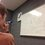# The Golden Ratio: Infinite PossibilitiesHere is the first post introducing the golden ratio.

What else is there to the Golden Ratio? We will see that there are infinite possibilities with it. Here are a few.

From the first notable equation mentioned in the previous post, we can take a square root of both sides. We just get another relation involving $\phi$. The left hand side is $\phi$ itself, but the right hand side is some other expression containing $\phi$. What happens if I substitute the $\phi$ on the right side with something else. I can then repeat. Here is the math:

$\phi$ $=\sqrt{1+\phi}$ $=\sqrt{1+\sqrt{1+\phi}}$ $=\sqrt{1+\sqrt{1+\sqrt{1+\phi}}}$ $=\sqrt{1+\sqrt{1+\sqrt{1+\sqrt{1+\sqrt{1+\cdots}}}}}$

We can extend this operation so that it goes on forever. This is what's called an infinite radical. But it still always equals the golden ratio. This number is starting to seem more special than just some ratio.

Now, using the second notable equation, let's apply the same idea:

$\phi$ $=1+\frac{1}{\phi}$ $=1+\frac{1}{1+\frac{1}{\phi}}$ $=1+\frac{1}{1+\frac{1}{1+\frac{1}{1+\frac{1}{1+\cdots}}}}$

This goes on forever too! And this one's called a continued fraction. Continued fractions are a well developed subject of mathematical study, where all the one's change to other numbers. Every number has it's own continued fraction. And, low and behold, the golden ratio has the simplest continued fraction of all.Note by Bob Krueger
7 years ago

This discussion board is a place to discuss our Daily Challenges and the math and science related to those challenges. Explanations are more than just a solution — they should explain the steps and thinking strategies that you used to obtain the solution. Comments should further the discussion of math and science.

When posting on Brilliant:

• Use the emojis to react to an explanation, whether you're congratulating a job well done , or just really confused .
• Ask specific questions about the challenge or the steps in somebody's explanation. Well-posed questions can add a lot to the discussion, but posting "I don't understand!" doesn't help anyone.
• Try to contribute something new to the discussion, whether it is an extension, generalization or other idea related to the challenge.

MarkdownAppears as
*italics* or _italics_ italics
**bold** or __bold__ bold
- bulleted- list
• bulleted
• list
1. numbered2. list
1. numbered
2. list
Note: you must add a full line of space before and after lists for them to show up correctly
paragraph 1paragraph 2

paragraph 1

paragraph 2

[example link](https://brilliant.org)example link
> This is a quote
This is a quote
    # I indented these lines
# 4 spaces, and now they show
# up as a code block.

print "hello world"
# I indented these lines
# 4 spaces, and now they show
# up as a code block.

print "hello world"
MathAppears as
Remember to wrap math in $$ ... $$ or $ ... $ to ensure proper formatting.
2 \times 3 $2 \times 3$
2^{34} $2^{34}$
a_{i-1} $a_{i-1}$
\frac{2}{3} $\frac{2}{3}$
\sqrt{2} $\sqrt{2}$
\sum_{i=1}^3 $\sum_{i=1}^3$
\sin \theta $\sin \theta$
\boxed{123} $\boxed{123}$

Sort by:

This is AWESOME !!!!!!!!

- 7 years ago

Nice..challenging!

- 7 years ago

Prove that 0! equals 1

- 7 years ago

How many ways are there to choose 0 objects from 0 objects?

One: DO NOTHING!

(I got this from AoPS. It's not really a rigorous proof of 0!=1 but it explains it well.)

- 7 years ago

nCn is equal to 1. so (n!)/(n-n)!.(n!) = 1 therefore (n-n)!=0! must be equal to 1.

- 7 years ago

(n-1)! * n = n! For n = 1: (1-1)! * 1 = 1! 0! *1 = 1 0! = 1

- 7 years ago

ncn=1 ,implies n!/(n-n)! .n!=1.. so,0!=1 hence proved..

- 7 years ago

Good..................

- 7 years ago

Can you prove these equation chains?

- 7 years ago

Because I derived them using facts about the golden ratio, there is no need to prove them. They are already proven.

- 7 years ago

prove a^0=1

- 7 years ago

See, Devansh.........$a^{b}*a^{-b}=a^{b-b}=a^{0}=a*\frac{1}{a}=1$

- 6 years, 10 months ago